Next: The FACES dataset Up: Density estimation experiments Previous: Digits and digit pairs

### The ALARM network

Our second set of density estimation experiments features the ALARM network as the data generating mechanism [Heckerman, Geiger, Chickering 1995,Cheng, Bell, Liu 1997]. This Bayesian network was constructed from expert knowledge as a medical diagnostic alarm message system for patient monitoring. The domain has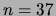discrete variables taking between 2 and 4 values, connected by 46 directed arcs. Note that this network is not a tree or a mixture of trees, but the topology of the graph is sparse, suggesting the possibility of approximating the dependency structure by a mixture of trees with a small number of components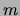. We generated a training set having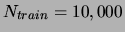data points and a separate test set of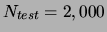data points. On these sets we trained and compared the following methods: mixtures of trees (MT), mixtures of factorial (MF) distributions, the true model, and gzip.'' For MT and MF the model orderand the degree of smoothing were selected by cross validation on randomly selected subsets of the training set.

 Model Train likelihood Test likelihood [bits/data point] [bits/data point] ALARM net 13.148 13.264 Mixture of trees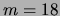13.51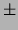0.04 14.550.06 Mixture of factorials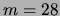17.110.12 17.640.09 Base rate 30.99 31.17 gzip 40.345 41.260

The results are presented in Table 3, where we see that the MT model outperforms the MF model as well as gzip and the base rate model.

 Model Train likelihood Test likelihood [bits/data point] [bits/data point] ALARM net 13.167 13.264 Mixture of trees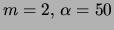14.560.16 15.510.11 Mixture of factorials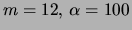18.200.37 19.990.49 Base rate 31.23 31.18 gzip 45.960 46.072

To examine the sensitivity of the algorithms to the size of the data set we ran the same experiment with a training set of size 1,000. The results are presented in Table 4. Again, the MT model is the closest to the true model. Notice that the degradation in performance for the mixture of trees is relatively mild (about 1 bit), whereas the model complexity is reduced significantly. This indicates the important role played by the tree structures in fitting the data and motivates the advantage of the mixture of trees over the mixture of factorials for this data set.Next: The FACES dataset Up: Density estimation experiments Previous: Digits and digit pairs
Journal of Machine Learning Research 2000-10-19